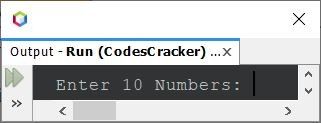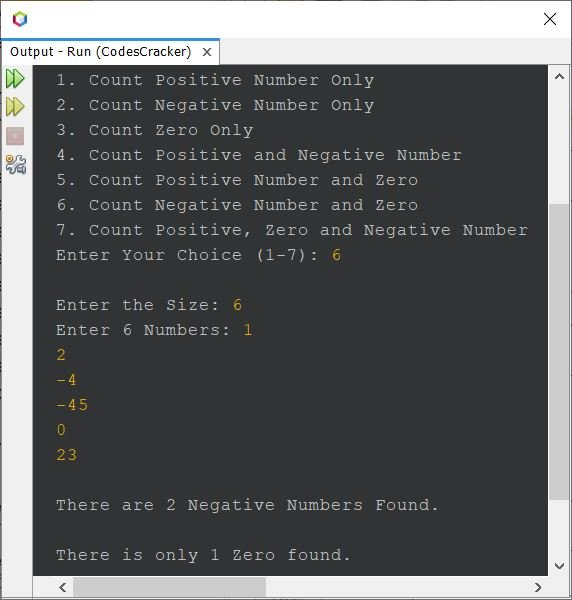# Java Program to Count Positive, Zero and Negative Numbers

## Count Positive, Negative and Zero in Java

To count the number of positive and negative numbers along with zero from a given set of numbers entered by user, you have to first receive some set of numbers say 10 numbers. Then check all the number using for loop one by one, to count the number of positive, zero, and negative number(s) available in the given set of 10 numbers and display the output on the screen as shown in the following program.

```import java.util.Scanner;

public class CodesCracker
{
public static void main(String[] args)
{
int countP=0, countN=0, countZ=0, i;
int[] arr = new int;
Scanner scan = new Scanner(System.in);

System.out.print("Enter 10 Numbers: ");
for(i=0; i<10; i++)
arr[i] = scan.nextInt();

for(i=0; i<10; i++)
{
if(arr[i]<0)
countN++;
else if(arr[i]>0)
countP++;
else
countZ++;
}

System.out.println("\nTotal Positive Number: " +countP);
System.out.println("Total Negative Number: " +countN);
System.out.println("Total Zero: " +countZ);
}
}```

When the above Java Program is compiled and executed, it will produce the following initial output:Now supply any 10 numbers. Here is its sample run with user input 32, 43, 0, -43, -54, -65, 23, 0, 53, 13The above program can also be created as:

```import java.util.Scanner;

public class CodesCracker
{
public static void main(String[] args)
{
int num, countP=0, countN=0, countZ=0;
Scanner scan = new Scanner(System.in);

System.out.print("Enter 10 Numbers: ");
for(int i=0; i<10; i++)
{
num = scan.nextInt();
if(num<0)
countN++;
else if(num>0)
countP++;
else
countZ++;
}

System.out.println("\nTotal Positive Number: " +countP);
System.out.println("Total Negative Number: " +countN);
System.out.println("Total Zero: " +countZ);
}
}```

## Count Positive and Negative Numbers in Java using while Loop

This program is similar to previous one. But it is created using while loop. Also the program allows user to define the size or the amount of numbers to enter.

```import java.util.Scanner;

public class CodesCracker
{
public static void main(String[] args)
{
int tot, num, i=0, countP=0, countN=0;
Scanner scan = new Scanner(System.in);

System.out.print("Enter the Size: ");
tot = scan.nextInt();
System.out.print("Enter " +tot+ " Numbers: ");
while(i<tot)
{
num = scan.nextInt();
if(num<0)
countN++;
else if(num>0)
countP++;
i++;
}

System.out.println("\nTotal Positive Number: " +countP);
System.out.println("Total Negative Number: " +countN);
}
}```

## Count Positive and Negative Numbers using an Array

The question is, write a Java program to count total number of positive and negative numbers available in an array of n numbers. The answer to this question, is the program given below:

```import java.util.Scanner;

public class CodesCracker
{
public static void main(String[] args)
{
int countP=0, countN=0;
Scanner scan = new Scanner(System.in);

System.out.print("Enter the Size: ");
int tot = scan.nextInt();
int[] nums = new int[tot];
System.out.print("Enter " +tot+ " Numbers: ");
for(int i=0; i<tot; i++)
{
nums[i] = scan.nextInt();
if(nums[i]<0)
countN++;
else if(nums[i]>0)
countP++;
}

System.out.println("\nTotal Positive Number: " +countP);
System.out.println("Total Negative Number: " +countN);
}
}```

## Mega Program on Counting Positive, Negative and Zero in Java

This is the last program of this article. I've called this program as the mega program of this article, because it includes all the options.

```import java.util.Scanner;

public class CodesCracker
{
public static void main(String[] args)
{
int num, countP=0, countN=0, countZ=0;
Scanner scan = new Scanner(System.in);

System.out.println("1. Count Positive Number Only");
System.out.println("2. Count Negative Number Only");
System.out.println("3. Count Zero Only");
System.out.println("4. Count Positive and Negative Number");
System.out.println("5. Count Positive Number and Zero");
System.out.println("6. Count Negative Number and Zero");
System.out.println("7. Count Positive, Zero and Negative Number");
int choice = scan.nextInt();

if(choice>=1 && choice<=7)
{
System.out.print("\nEnter the Size: ");
int tot = scan.nextInt();
System.out.print("Enter " +tot+ " Numbers: ");
if(choice==1)
{
for(int i=0; i<tot; i++)
{
num = scan.nextInt();
if(num>0)
countP++;
}
}
else if(choice==2)
{
for(int i=0; i<tot; i++)
{
num = scan.nextInt();
if(num<0)
countN++;
}
}
else if(choice==3)
{
for(int i=0; i<tot; i++)
{
num = scan.nextInt();
if(num==0)
countZ++;
}
}
else if(choice==4)
{
for(int i=0; i<tot; i++)
{
num = scan.nextInt();
if(num>0)
countP++;
else if(num<0)
countN++;
}
}
else if(choice==5)
{
for(int i=0; i<tot; i++)
{
num = scan.nextInt();
if(num>0)
countP++;
else if(num==0)
countZ++;
}
}
else if(choice==6)
{
for(int i=0; i<tot; i++)
{
num = scan.nextInt();
if(num<0)
countN++;
else if(num==0)
countZ++;
}
}
else if(choice==7)
{
for(int i=0; i<tot; i++)
{
num = scan.nextInt();
if(num>0)
countP++;
else if(num<0)
countN++;
else
countZ++;
}
}
if(countP!=0)
{
if(countP==1)
System.out.println("\nThere is only 1 Positive Number Found.");
else
System.out.println("\nThere are " +countP+ " Positive Numbers Found.");
}
if(countN!=0)
{
if(countN==1)
System.out.println("\nThere is only 1 Negative Number Found.");
else
System.out.println("\nThere are " +countN+ " Negative Numbers Found.");
}
if(countZ!=0)
{
if(countZ==1)
System.out.println("\nThere is only 1 Zero found.");
else
System.out.println("\nThere are " +countZ+ " Zero Found.");
}
}
else
System.out.println("\nInvalid Input!");
}
}```

Here is its sample run with user input 6 as choice to count negative number and zero only, 6 as size to count based on 6 numbers, 1, 2, -4, -45, 0, 23 as six numbers:#### Same Program in Other Languages

Java Online Test

« Previous Program Next Program »